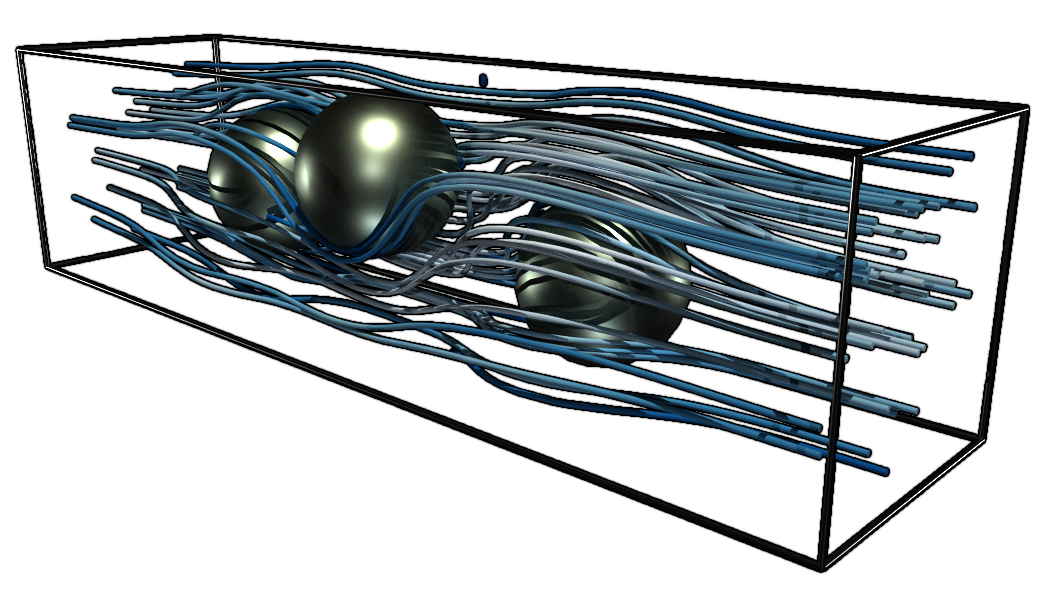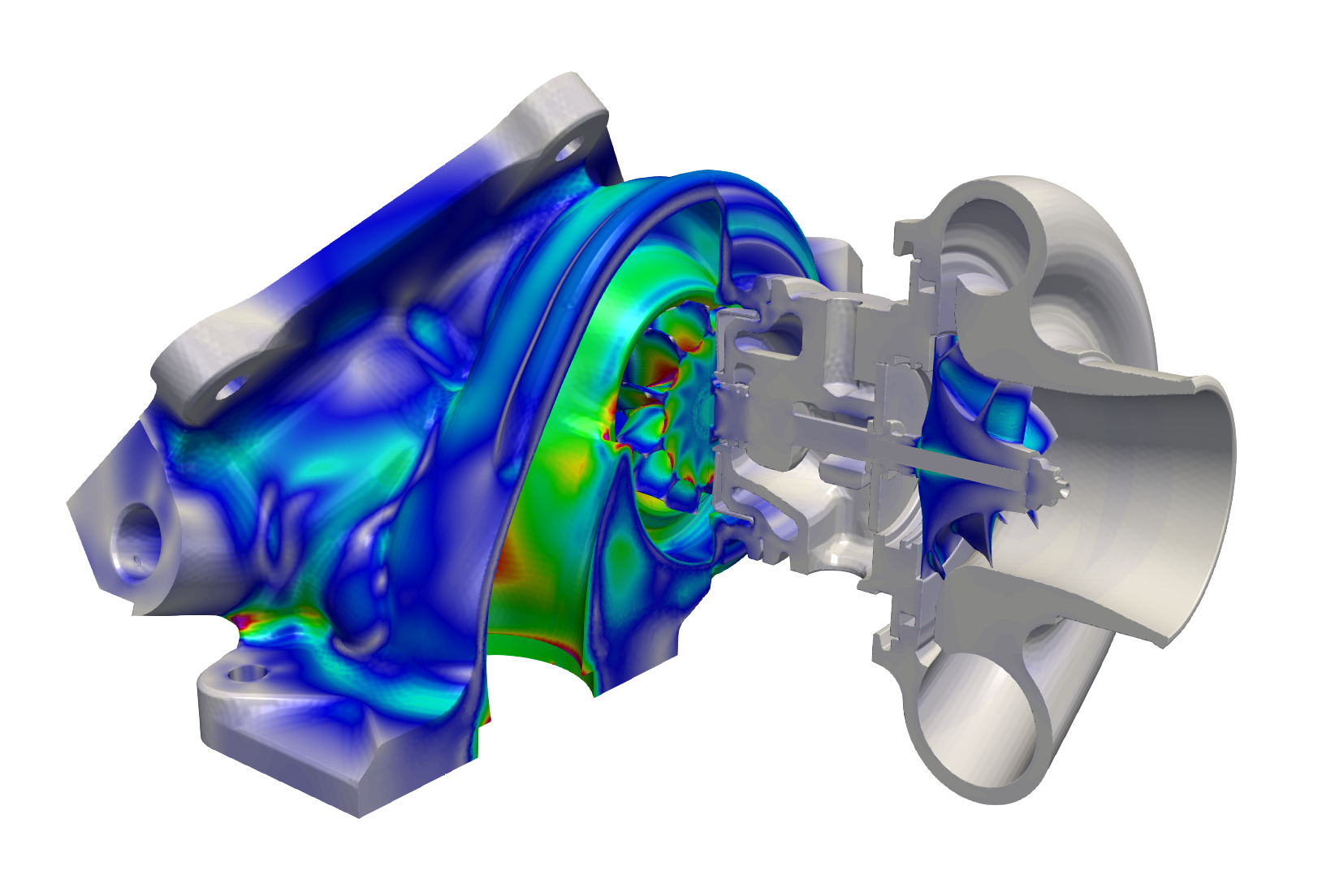# FEniCSx

## The FEniCS computing platform

FEniCS is a popular open-source (LGPLv3) computing platform for solving partial differential equations (PDEs). FEniCS enables users to quickly translate scientific models into efficient finite element code. With the high-level Python and C++ interfaces to FEniCS, it is easy to get started, but FEniCS offers also powerful capabilities for more experienced programmers. FEniCS runs on a multitude of platforms ranging from laptops to high-performance clusters.

## Solving a PDE in FEniCS

As an illustration of how to program a simple PDE model with FEniCS, consider the Stokes equations in variational form:

$\int_{\Omega} \mathrm{grad} \, u : \mathrm{grad} \, v \,\mathrm{d}x \, - \int_{\Omega} p \, \mathrm{div} \, v \,\mathrm{d}x + \int_{\Omega} \mathrm{div} \, u \, q \,\mathrm{d}x = \int_{\Omega} f \cdot v \,\mathrm{d}x.$

The variational problem is easily transcribed into Python using mathematical operators in FEniCS:

# Define function space
P2 = VectorElement('P', tetrahedron, 2)
P1 = FiniteElement('P', tetrahedron, 1)
TH = P2 * P1
W = FunctionSpace(mesh, TH)

# Define variational problem
(u, p) = TrialFunctions(W)
(v, q) = TestFunctions(W)
L = dot(f, v)*dx

# Compute solution
w = Function(W)
solve(a == L, w, [bc1, bc0])


The above code snippet also shows how to define a suitable finite element function space, using continuous piecewise quadratic vector-valued functions for the velocity and continuous piecewise linear functions for the pressure (Taylor-Hood). The computational domain and mesh are also easily created with FEniCS, here defined by three spheres immersed in a 3D channel.# Define domain
h = 0.25
r = 0.3*h
box = Box(Point(0, 0, 0), Point(1, h, h))
s0 = Sphere(Point(0.3, 0.50*h, 0.50*h), r)
s1 = Sphere(Point(0.5, 0.65*h, 0.65*h), r)
s2 = Sphere(Point(0.7, 0.35*h, 0.35*h), r)
domain = box - s0 - s1 - s2

# Generate mesh
mesh = generate_mesh(domain, 32)


## High-performance computingEach component of the FEniCS platform has been fundamentally designed for parallel processing. Executing a FEniCS script in parallel is as simple as calling mpirun -np 64 python script.py. This framework allows for rapid prototyping of finite element formulations and solvers on laptops and workstations, and the same code may then be deployed on large high-performance computers.

The figure shows the von Mises stresses computed from a nonlinear thermomechanical FEniCS simulation of a turbocharger. The finite element system of linear equations comprises more than $$3.3 \times 10^9$$ degrees of freedom. The solver was initially developed on a desktop computer for a small scale problem, and the same code was then deployed on a supercomputer using over 24000 parallel processes.

## Installation and documentation

FEniCS is available for a range of platforms (Linux, Mac, Windows). Choose between Docker containers, binary packages and source code. Visit our installation page to get the latest version of FEniCS. FEniCS comes with extensive documentation and numerous examples. A good starting point is the FEniCS Tutorial.